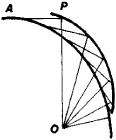# Pedal Curve

(redirected from Contrapedal curve)
Also found in: Dictionary.

## pedal curve

[′ped·əl ‚kərv]
(mathematics)
The pedal curve of a given curve C with respect to a fixed point P is the locus of the foot of the perpendicular from P to a variable tangent to C. Also known as first pedal curve; first positive pedal curve; positive pedal curve.
Any curve that can be derived from a given curve C by repeated application of the procedure specified in the first definition.

## Pedal Curve

(or pedal). The locus P of the feet of the perpendiculars dropped from a point O to the tangents of a given curve A is called the pedal curve of A with respect to the point 0. An example of a pedal curve is given in Figure 1. The curve A is said to be the first negative pedal curve of P with respect to the point 0.Figure 1

Site: Follow: Share:
Open / Close